Next: Free-fall under gravity Up: Motion in 1 dimension Previous: Motion with constant velocity

## Motion with constant acceleration

Motion with constant acceleration occurs in everyday life whenever an object is dropped: the object moves downward with the constant acceleration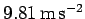, under the influence of gravity.

Fig. 8 shows the graphs of displacement versus time and velocity versus time for a body moving with constant acceleration. It can be seen that the displacement-time graph consists of a curved-line whose gradient (slope) is increasing in time. This line can be represented algebraically as(19)

Here,is the displacement at time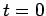: this quantity can be determined from the graph as the intercept of the curved-line with the-axis. Likewise,is the body's instantaneous velocity at time.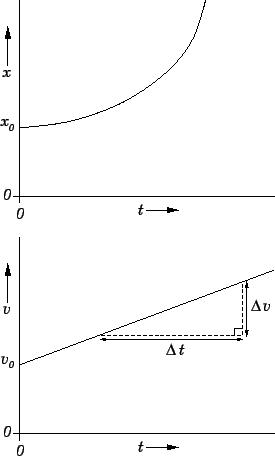The velocity-time graph consists of a straight-line which can be represented algebraically as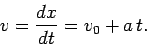(20)

The quantityis determined from the graph as the intercept of the straight-line with the-axis. The quantityis the constant acceleration: this can be determined graphically as the gradient of the straight-line (i.e., the ratio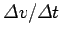, as shown). Note that, as expected.

Equations (19) and (20) can be rearranged to give the following set of three useful formulae which characterize motion with constant acceleration: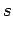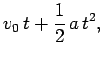(21)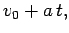(22)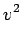(23)

Here,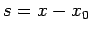is the net distance traveled afterseconds.

Fig. 9 shows a displacement versus time graph for a slightly more complicated case of accelerated motion. The body in question accelerates to the right [since the gradient (slope) of the graph is increasing in time] between timesand. The body then moves to the right (sinceis increasing in time) with a constant velocity (since the graph is a straight line) between timesand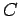. Finally, the body decelerates [since the gradient (slope) of the graph is decreasing in time] between timesand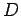.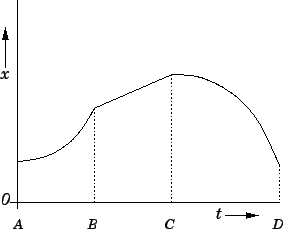Next: Free-fall under gravity Up: Motion in 1 dimension Previous: Motion with constant velocity
Richard Fitzpatrick 2006-02-02